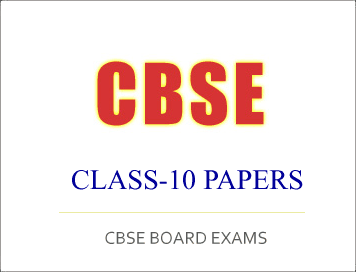# CBSE Class-10 Exam 2019 : All India Scheme Question Paper, Mathematics

Disclaimer: This website is NOT associated with CBSE, for official website of CBSE visit - www.cbse.gov.in## CBSE Class-10 Exam 2019 :  Mathematics

General Instructions :

(i) All questions are compulsory.
(ii) The question paper consists of 30 questions divided into four sections A, B, C and D.
(iii) Section A contains 6 questions of 1 mark each. Section B contains 6 questions of 2 marks each, Section C contains 10 questions of 3 marks each and Section D contains 8 questions of 4 marks each.
(iv) There is no overall choice. However, an internal choice has been provided in two questions of 1 mark each, two questions of 2 marks each, four questions of 3 marks each and three questions of 4 marks each. You have to attempt only one of the alternatives in all such questions.
(v) Use of calculators is not permitted